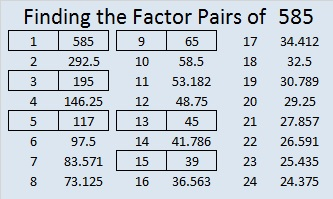What can you see in the number 585?

This week I watched an excellent video titled 5 x 9 is more than 45. Indeed 45 is so much more than simply 5 x 9. Every multiplication fact is much more than that mere fact, but Steve Wyborney used 5 x 9 = 45 in his video… Guess what! 585 is a multiple of 45.

As I thought about the number 585, I marveled at some of the hidden mysteries this number holds.

Since 585 is divisible by two different centered square numbers, 5 and 13, I saw that 585 could be represented by this lovely array that has 45 larger squares made up of 13 smaller colorful squares. When you look at the array, do you just see 585 squares or can you see even more multiplication and division facts? If you rotate the array 90 degrees, do the facts change?What do you see in this array of 117 medium sized squares made up of 5 smaller squares:Or this more simple looking array of sixty-five 3 x 3 squares.All of these arrays are in just two dimensions. A 5 x 9 x 13 rectangular prism is ONE way to represent 585 in three dimensions.

—————————————————————————————————

Since factors 5, 13, and 65 are hypotenuses of primitive Pythagorean triples, 585 is the hypotenuse of four Pythagorean triples. Each triple has a different greatest common factor. Can you figure out what each one is?

• 144-567-585
• 225-540-585
• 297-504-585
• 351-468-585

—————————————————————————————————

And if all of that wasn’t enough, Stetson.edu informs us that 585 is a palindrome in 3 different bases!

• 585 = 1001001001 in base 2
• 585 = 1111 in base 8
• 585 = 585 in base 10

Here are two different methods of determining 585 in base 8. In both methods the base 8 representation is in blue.—————————————————————————————————

• 585 is a composite number.
• Prime factorization: 585 = 3 x 3 x 5 x 13, which can be written 585 = (3^2) x 5 x 13
• The exponents in the prime factorization are 2, 1, and 1. Adding one to each and multiplying we get (2 + 1)(1 + 1)(1 + 1) = 3 x 2 x 2 = 12. Therefore 585 has exactly 12 factors.
• Factors of 585: 1, 3, 5, 9, 13, 15, 39, 45, 65, 117, 195, 585
• Factor pairs: 585 = 1 x 585, 3 x 195, 5 x 117, 9 x 65, 13 x 45, or 15 x 39
• Taking the factor pair with the largest square number factor, we get √585 = (√9)(√65) = 3√65 ≈ 24.186771.Joseph Nebus